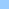# bits_and_byte

how to analyse the byte into bits
bit byte modbits and byte ```> What I need to do is analyse the byte into bits, but I'm not certian >as to how to go about doing this. Are there boolean controls in lingo >that can do this? Hi Shane, I assume that your byte comes in as an integer, or that you can convert it to an integer somehow. If so, you can use the "mod" operator to look at the last bit, and the "/" operator to chop the last bit off so that you can look at the next one. Here's a handler that reads the bits from right to left: on treatBitsIn byte set bitWeight = 1 repeat while byte set theBit = byte mod 2 -- do something with theBit and bitWeight set bitWeight = bitWeight * 2 set byte = byte / 2 end repeat end And here are a couple of bit functions which work with integers: on setBit myInteger, theBit, bitValue set magnitude = integer (power (2, theBit-1)) set myInteger = (((myInteger / magnitude / 2) * 2 + bitValue) * ¬ magnitude) + myInteger mod magnitude return myInteger end setBit on getBit myInteger, theBit return (myInteger / integer (power (2, theBit-1))) mod 2 end getBit Is this of any help? Cheers, James -- James Newton Tel: + 33 3 80 75 16 69 ```Home shock + cgi Bits 'n pieces Director Lingo ShockLets Contact